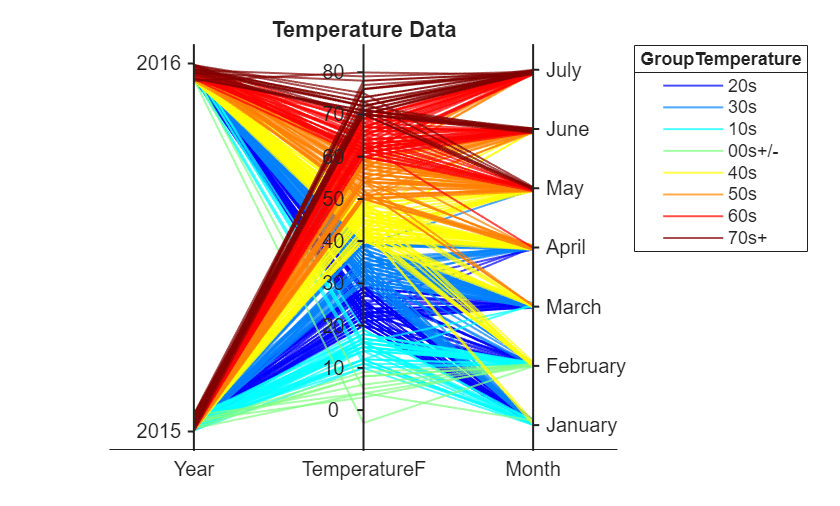# 使用平行坐标图探索表数据

### 以表的形式导入文件

```tbl = readtable('TemperatureData.csv'); head(tbl)```
```ans=8×4 table Year Month Day TemperatureF ____ ___________ ___ ____________ 2015 {'January'} 1 23 2015 {'January'} 2 31 2015 {'January'} 3 25 2015 {'January'} 4 39 2015 {'January'} 5 29 2015 {'January'} 6 12 2015 {'January'} 7 10 2015 {'January'} 8 4 ```

### 创建基本的平行坐标图

`parallelplot(head(tbl))`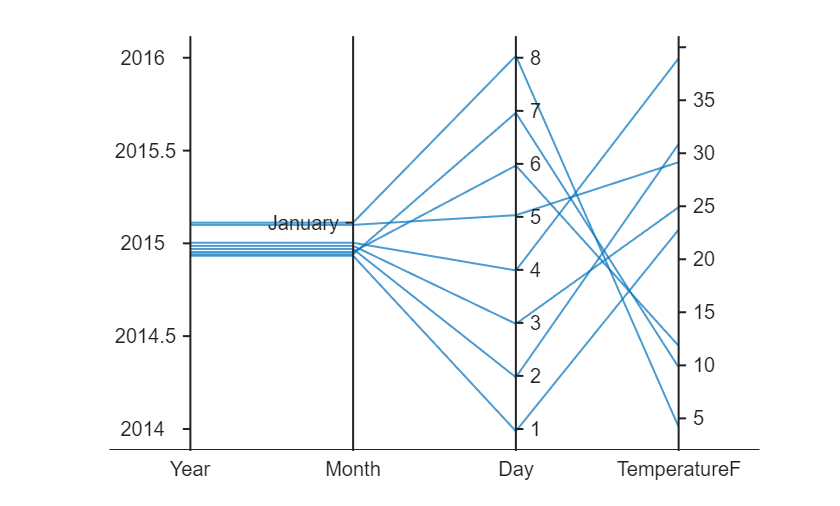`tbl.Year = categorical(tbl.Year);`

`p = parallelplot(tbl)`
```p = ParallelCoordinatesPlot with properties: SourceTable: [565x4 table] CoordinateVariables: {'Year' 'Month' 'Day' 'TemperatureF'} GroupVariable: '' Show all properties ```
`p.Title = 'Temperature Data';`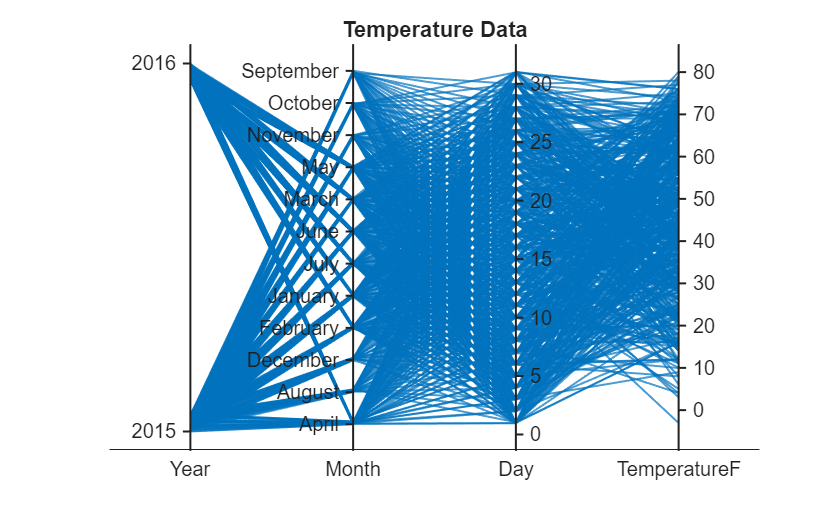### 对绘图线条分组

`p.GroupVariable = 'Year';`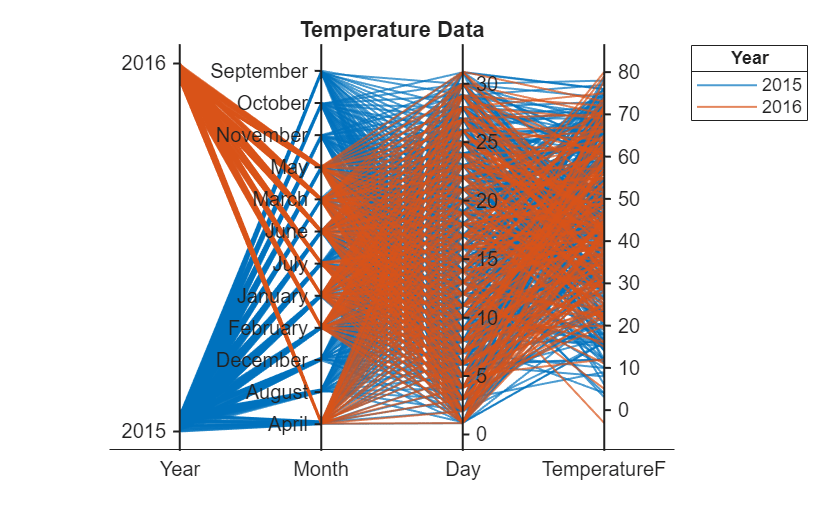### 交互式重排坐标变量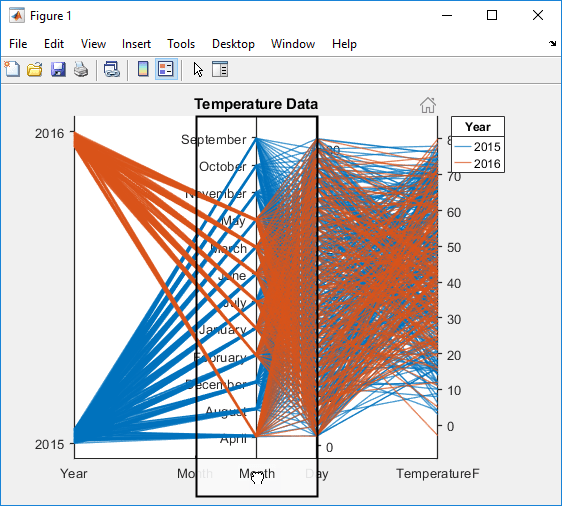### 选择部分坐标变量

`p.CoordinateVariables = [1 4 2];`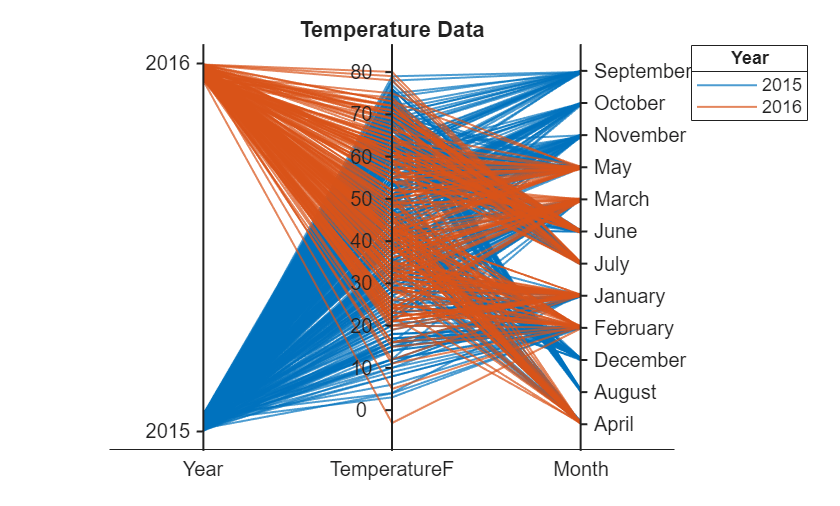### 修改坐标变量中的类别

```uniqueMonth = {'September','October','November','December','August'}; uniqueMonthIdx = ismember(p.SourceTable.Month,uniqueMonth); p.SourceTable(uniqueMonthIdx,:) = [];``````categoricalMonth = categorical(p.SourceTable.Month); newOrder = {'January','February','March','April','May','June','July'}; orderMonth = reordercats(categoricalMonth,newOrder); p.SourceTable.Month = orderMonth;```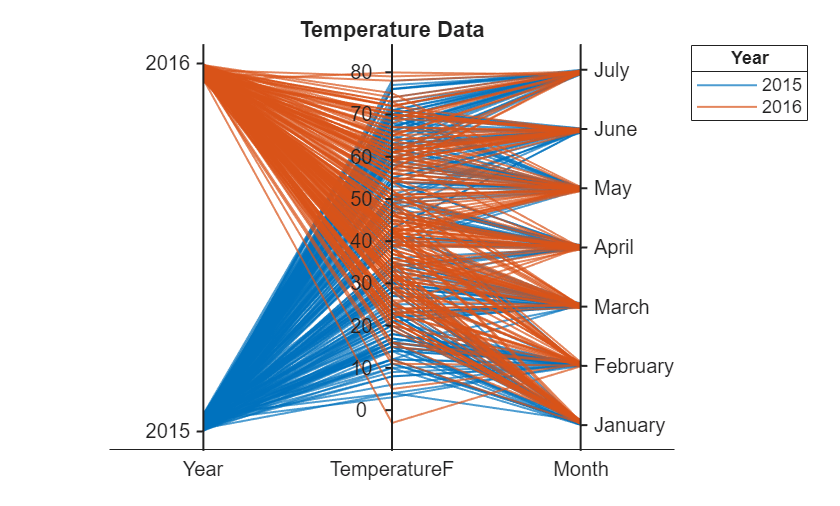### 基于分 bin 后的值对绘图线条分组

`min(p.SourceTable.TemperatureF)`
```ans = -3 ```
`max(p.SourceTable.TemperatureF)`
```ans = 80 ```
```binEdges = [-3 10:10:80]; bins = {'00s+/-','10s','20s','30s','40s','50s','60s','70s+'}; groupTemperature = discretize(p.SourceTable.TemperatureF, ... binEdges,'categorical',bins);```

```p.SourceTable.GroupTemperature = groupTemperature; p.GroupVariable = 'GroupTemperature';```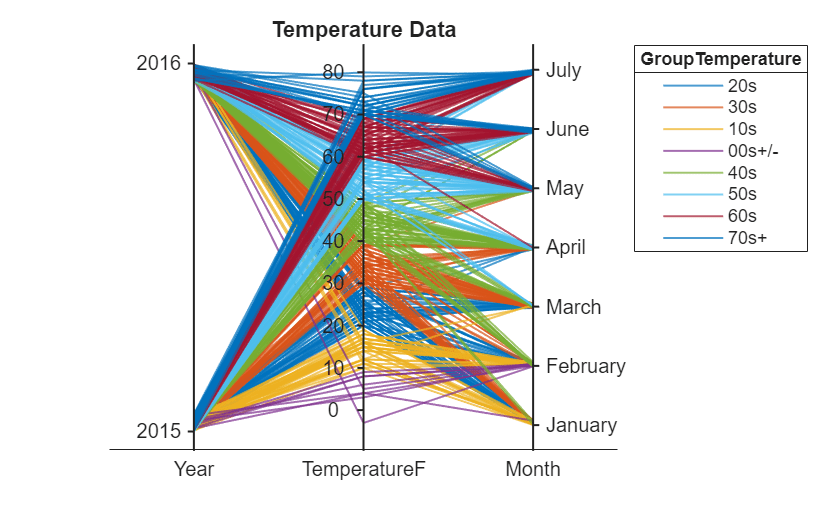`p.Color = jet(8);`